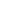Math Worksheets

• Detail View
• List View

Long Division Worksheets (with and without Remainders)

Worksheets of long division (with and without Remainders)

Mixed Division Worksheets (with and without Remainders)

Worksheets of mixed division (with and without Remainders)

Single Digit Quotient Simple Division Worksheets (with Remainders)

Worksheets of single digit quotient division (with Remainders)

Single Digit Quotient Simple Division Worksheets (No Remainders)

Worksheets of single digit quotient division (with no Remainders)

Visual Division Worksheets (With Remainders)

Worksheets of visual division (with Remainders)

Visual Division Worksheets (No Remainders)

Worksheets of visual division (no Remainders)

Double Digit Multiplication Worksheets

Worksheets of double digit multiplication

Single Digit Simple Multiplication Worksheets

Worksheets of single digit multiplication

Multiplication Times Tables Worksheets

Worksheets of times tables (multiplication 1 to 12)

Visual Multiplication Worksheets

Worksheets of visual multiplication

Double Digit Subtraction Worksheets (With Borrowing)

Worksheets of double digit subtraction (with borrowing)

Double Digit Subtraction Worksheets (No Borrowing)

Worksheets of double digit subtraction (no borrowing)